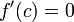# Higher derivative test is conclusive for locally analytic function

Suppose$f$ is a function,$c$ is a point in the interior of the domain of$f$, and$f$ is analytic about$c$, i.e., there is a power series centered at$c$ that converges to$f$ on an open interval containing$c$. In particular, this means that$f$ is infinitely differentiable at$c$. The assumption of$c$ being a critical point also forces$f'(c) = 0$.
In this case, the higher derivative test must always be conclusive for$f$ at$c$.